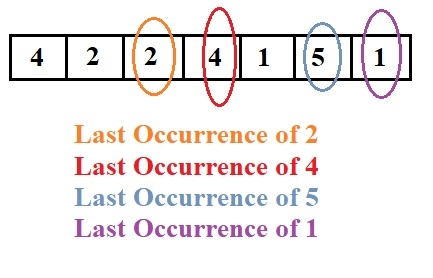# Print the last occurrence of elements in array in relative order in C Program.

Given an array a[] with elements and the task is to print the last occurrences of the given elements in the list. Here we not only have to remove the duplicate elements but also we have to maintain the order of the occurrences of the elements in an array as per the last time they have occurred.

Like we have an array of 6 elements also containing some duplicate values i.e., {1,3, 2, 3, 1, 2} so the result should be in form of 3 1 2.

## Example

Input: a[]={4,2,2,4,1,5,1}
Output : 2 4 5 1## Algorithm

START
Step 1-> Declare function void printelements(int a[], int n)
Use STL unordered_map<int, int> ele
Loop For int i=0 and i<n and i++
Set ele[a[i]]=i
Loop For int i=0 and i<n and i++
IF ele[a[i]]=i
Print a[i]
End
End
Step 2 -> main()
Declare array a[]={4,2,2,4,1,5,1}
Declare int n=sizeof(a)/sizeof(a)
Call Function printelements(a,n)
STOP

## Example

#include <bits/stdc++.h>
using namespace std;
void printelements(int a[], int n) {
unordered_map<int, int> ele;
for (int i = 0; i < n; i++)
ele[a[i]] = i;
for (int i = 0; i < n; i++) {
if (ele[a[i]] == i)
cout << a[i] << " ";
}
}
int main() {
int a[] = { 4,2,2,4,1,5,1 };
int n = sizeof(a) / sizeof(a);
printelements(a, n);
return 0;
}

## Output

if we run above program then it will generate following output

2 4 5 1